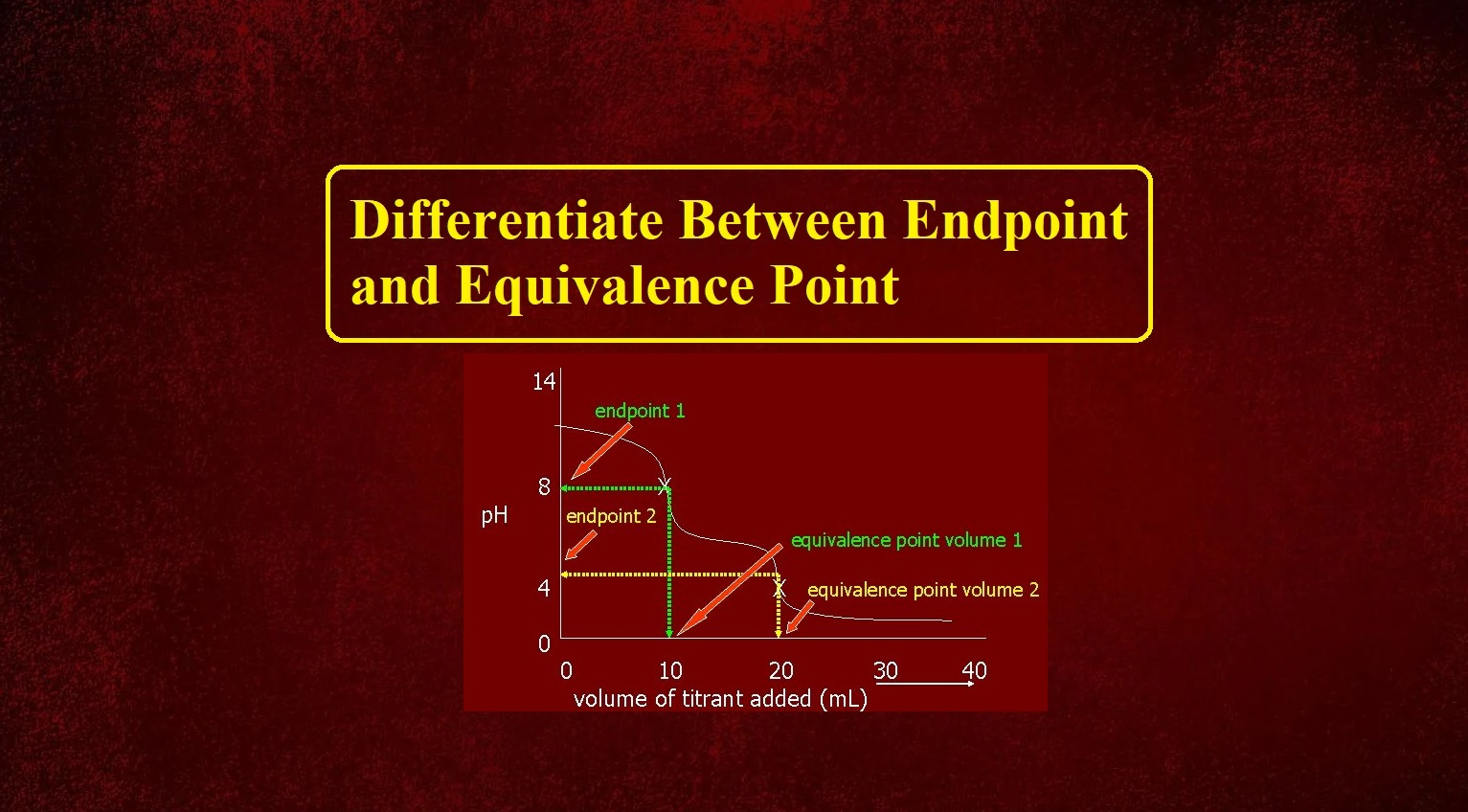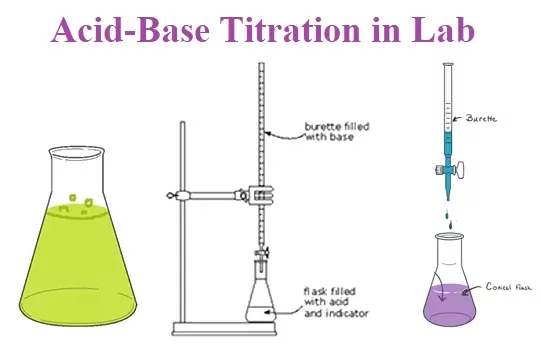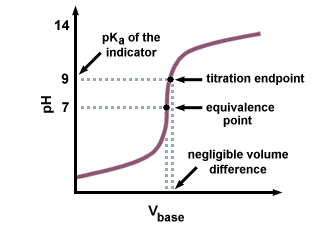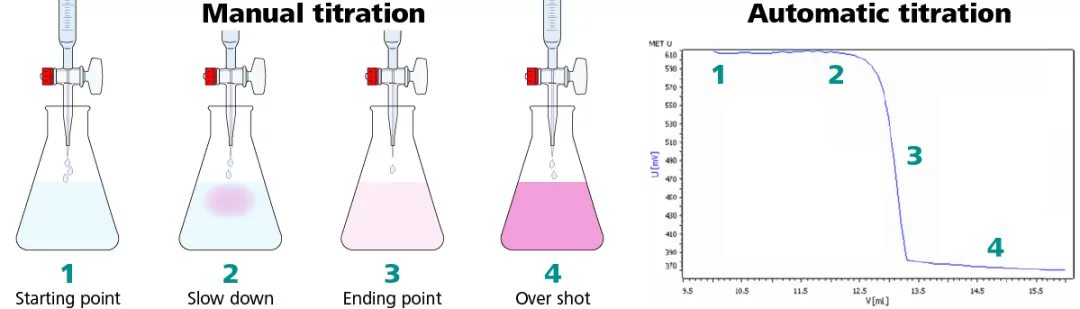# Differentiate Between Endpoint and Equivalence Point# Endpoint and Equivalence Point

Endpoint and Equivalence Point

Titration is a common laboratory method or process of determining the concentration of unknown solutions by using solutions of known concentration. Solution of known concentration is known as Titrant while the solution of unknown concentration is known as Analyte in titration technique. It is also known as titrimetric and volumetric analysis. The process is usually carried out by gradually adding a standard solution (i.e., a solution of known concentration) of titrating reagent, or titrant, from a burette, essentially a long graduated measuring tube with a stopcock and a delivery tube at its lower end. The addition is stopped when the equivalence point is reached.Endpoint and Equivalence Point

The equivalence point is the point in a titration where the amount of titrant added is enough to completely neutralize the analyte solution. The moles of titrant (standard solution) equal the moles of the solution with unknown concentration. For an acid-base reaction, it is also known as the Stoichiometric point because it is where the moles of acid are equal to the moles of base needed to neutralize the reaction.The endpoint is a point marking the completion of a process or a point in a titration at which a definite effect (such as a color change) is observed.
The endpoint also demonstrates the equivalence point, typically by some form of indicator. For example, with a color indicator, the solution changes color when the titration reaches its endpoint.Endpoint and Equivalence Point

 Equivalence Point Endpoint The equivalence point is the exact point in a titration when moles of one titrant equal the moles of the substance being titrated. The endpoint is the point at which the indicator changes color in a colorimetric titration. It is the point where the analyte has completely reacted with the titrant. It doesn’t always give the point where the analyte has completely reacted with the titrant. It does not indicate the completion of titration. It is obtained when titration is complete. It is not always indicated by colour change of the reaction mixture. It is always indicated by the color change of the reaction mixture. Weak acids can have multiple equivalence point Weak acids can have only one endpoint Comes before the endpoint. Comes after the equivalence point.

Endpoint and Equivalence Point